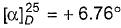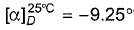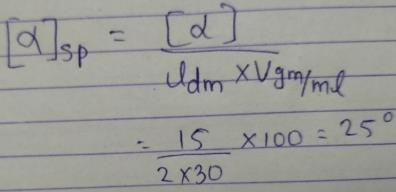Courses

# Test: Optical Isomerism Polarimetry (Isomerism)

## 12 Questions MCQ Test Chemistry Class 11 | Test: Optical Isomerism Polarimetry (Isomerism)

Description
This mock test of Test: Optical Isomerism Polarimetry (Isomerism) for Class 11 helps you for every Class 11 entrance exam. This contains 12 Multiple Choice Questions for Class 11 Test: Optical Isomerism Polarimetry (Isomerism) (mcq) to study with solutions a complete question bank. The solved questions answers in this Test: Optical Isomerism Polarimetry (Isomerism) quiz give you a good mix of easy questions and tough questions. Class 11 students definitely take this Test: Optical Isomerism Polarimetry (Isomerism) exercise for a better result in the exam. You can find other Test: Optical Isomerism Polarimetry (Isomerism) extra questions, long questions & short questions for Class 11 on EduRev as well by searching above.
QUESTION: 1

### Direction (Q. Nos. 1 - 8) This section contains 8 multiple choice questions. Each question has four choices (a), (b), (c) and (d), out of which ONLY ONE option is correct. Q. Which is true regarding a plane polarised light?

Solution:

The correct answer is Option C.
As it propagates, both magnitude of electric vector and direction of polarization varies.

QUESTION: 2

### At a given temperature and for a given wavelength of plane polarised light, the fundamental property of an optically active substance is

Solution:

The correct answer is Option C.
For a pure enantiomer, signs of specific rotation and its magnitude are fundamental properties as they do not depend on concentration of sample or length of sample tube.

QUESTION: 3

### What is the percentage composition of an enantiomeric mixture of 2-butanol with a specific rotation? Specific rotation of pure enantiomer (+)-2-butanol is +13.52°.

Solution:

Optical purity = optical rotation of mixture/optical rotation of pure compound .
(mixture) [α]D25  = +6.76°
+[α]D = +13.57°
Since rotation of two enantiomers cancel each other, so rotation is due to enantiomeric excess.
Enantiomeric excess = observed specific rotation/max. Specific rotation = 6.76/13.52×100
= 50%
Therefore, %age of each enantiomer is given by,
If we have (+) (-) mixture(+) is in excess,
%(+) = ee/2 + 50%
= 75 % (s)
%(-) = 25% (s)

QUESTION: 4

Which of the following is a comparatively significant factor affecting the magnitude of specific optical rotation?

Solution:

The correct answer is Option C.
The specific rotation of a molecule is the rotation in degrees observed upon passing polarized light through a path length of 1 decimetre (dm) at a concentration of 1 g/mL. Specific rotation is almost always reported along with the temperature, wavelength of light used, the solvent, and the concentration, since it is sensitive to these factors as well.
Magnitude of specific rotation depends on temperature. However, it does not depend on concentration, purity or length of sample tude.

QUESTION: 5

What can be said with certainty if a compound has?

Solution:

The correct answer is Option C.

A meso compound is optically inactive with zero specific rotation.

QUESTION: 6

If solution of a compound (30 g/100 mL of solution) has measured rotation of +15° in a 2 dm long sample tube, the specific rotation of this compound is

Solution:Where [α] = measured rotation, [α]sp = specific rotation, l = length of tube (in decimeter)

QUESTION: 7

Enantiomers are

Solution:

Enantiomers are chiral molecules that are mirror images of one another. Furthermore, the molecules are non-superimposable on one another. This means that the molecules cannot be placed on top of one another and give the same molecule. Chiral molecules with one or more stereocenters can be enantiomers.

QUESTION: 8

Which of the following is not true of enantiomers ? They have the same

Solution:

Specific rotation ([α]) is a property of a chiral chemical compound. It is defined as the change in orientation of monochromatic plane-polarized light, per unit distance–concentration product, as the light passes through a sample of a compound in solution.

*Multiple options can be correct
QUESTION: 9

Direction (Q. Nos. 9-12) This section contains 4 multiple choice questions. Each question has four
choices (a), (b), (c) and (d), out of which ONE or  MORE THANT ONE  is correct.

Q.

Specification rotation of an optically active isomer depends on

Solution:

For an optically active substance, defined by [α]θλ = α/γl, where α is the angle through which plane polarized light is rotated by a solution of mass concentration γ and path length l. Here θ is the Celsius temperature and λ the wavelength of the light at which the measurement is carried out.
Values for specific rotation are reported in units of deg·mL·g−1·dm−1, which are typically shortened to just degrees, wherein the other components of the unit are tacitly assumed. These values should always be accompanied by information about the temperature, solvent and wavelength of light used, as all of these variables can affect the specific rotation. As noted above, temperature and wavelength are frequently reported as a superscript and subscript, respectively, while the solvent is reported parenthetically, or omitted if it happens to be water.

*Multiple options can be correct
QUESTION: 10

A solution has a measured optical rotation of —24° at 25°C using D-line of sodium lamp. What can be predicted regarding this solution?

Solution:

Option A, B and C are correct. For this case, there are 3 possibilities. Either the solution is itself laboratory, or its laevo + dextro with laevo in excess so that we have rotation of light in -. If B is true, then option c is also true. As (+) and (-) are two optical compounds that are not isomers. However, Option D is incorrect as optical rotation is independent of configuration of molecule.

*Multiple options can be correct
QUESTION: 11

The correct statement regarding a pair of enantiomers is/are

Solution:
*Multiple options can be correct
QUESTION: 12

What is/are true regarding Fischer projection of an optically active isomer?

Solution: